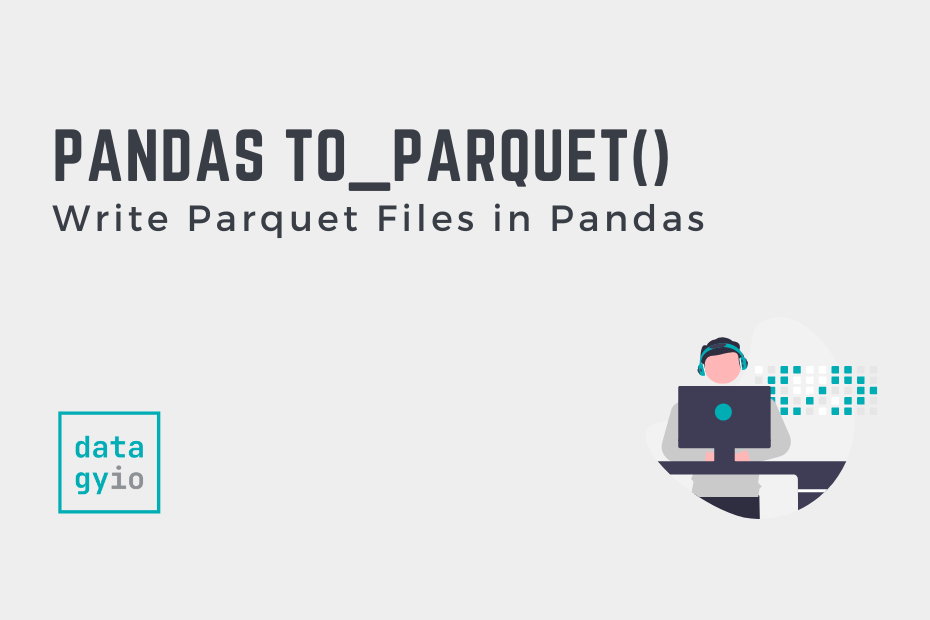# pd.to_parquet: Write Parquet Files in PandasIn this tutorial, you’ll learn how to use the Pandas to_parquet method to write parquet files in Pandas. While CSV files may be the ubiquitous file format for data analysts, they have limitations as your data size grows. This is where Apache Parquet files can help!

Want to learn how to read a parquet file in Pandas instead? Check out this comprehensive guide to reading parquet files in Pandas.

By the end of this tutorial, you’ll have learned:

• What Apache Parquet files are
• How to write parquet files with Pandas using the `pd.to_parquet()` method
• How to speed up writing parquet files with PyArrow
• How to specify the engine used to write a parquet file in Pandas

## What are Apache Parquet Files?

The Apache Parquet format is a column-oriented data file format. This means data are stored based on columns, rather than by rows. The benefits of this include significantly faster access to data, especially when querying only a subset of columns. This is because only particular can be read, rather than entire records.

The format is an open-source format that is specifically designed for data storage and retrieval. Because of this, its encoding schema is designed for handling massive amounts of data, especially spread across different files.

## Understanding the Pandas to_parquet Method

Before diving into using the Pandas `to_parquet()` method, let’s take a look at the different parameters and default arguments of the method. This will give you a strong understanding of the method’s abilities.

Let’s take a look at the `pd.to_parquet()` method:

``````# Understanding the Pandas read_parquet() Method
import pandas as pd
df = pd.DataFrame()
df.to_parquet(path, engine='auto', compression='snappy', index=None, partition_cols=None, **kwargs)``````

We can see that the method offers 5 parameters, 4 of which have default arguments provided. The table below breaks down the method’s parameters and provides descriptions of how they can be used.

Now that you have a good understanding of the various parameters available in the Pandas `to_parquet()` method, let’s dive into how to

## How to Write to a Parquet File with to_parquet

In order to write a Pandas DataFrame, you simply need to apply the `.to_parquet()` method to the DataFrame and pass in a path to where you want to save the file. Let’s take a look at how we can load a sample DataFrame and write it to a parquet file:

``````# Write a Pandas DataFrame to a Parquet File
import pandas as pd

df = pd.DataFrame({
'ID': range(5),
'Name':['Nik', 'Kate', 'Noelle', 'Autumn', 'Many'],
'Age': [33, 34, 27, 45, 23]
})

df.to_parquet('sample.parquet')``````

When you run the code above, a parquet file is created containing the DataFrame `df`.

## Use Compression When Writing a DataFrame to Parquet

The Pandas `to_parquet()` function also allows you to apply compression to a parquet file. By default, Pandas will use snappy compression. However, we can also use different formats, including gzip and brotli.

Let’s take a look at how we can use the `compression=` parameter to apply gzip compression to our DataFrame’s resulting parquet file:

``````# Apply gzip Compression to a Parquet File in Pandas
import pandas as pd

df = pd.DataFrame({
'ID': range(5),
'Name':['Nik', 'Kate', 'Noelle', 'Autumn', 'Many'],
'Age': [33, 34, 27, 45, 23]
})

df.to_parquet('df.parquet.gzip', compression='gzip') ``````

In the example above, we changed two pieces from our previous code:

1. We added `.gzip` to our file’s extension and
2. We passed in `compression='gzip'` into our method call

In the final section below, let’s take a look at how we can include the index when writing a DataFrame to a parquet file.

## Include the Index When Writing a DataFrame to Parquet

Similar to other Pandas methods to write a DataFrame to a file, including an index is easy. In order to do this, you can simply pass in `index=True` into the method. While the sample DataFrame’s index isn’t meaningful, this can be much more helpful when your index has more information.

Let’s take a look at how we can include the index when writing a DataFrame to a parquet file:

``````# Include an Index When Writing a DataFrame to a Parquet File
import pandas as pd

df = pd.DataFrame({
'ID': range(5),
'Name':['Nik', 'Kate', 'Noelle', 'Autumn', 'Many'],
'Age': [33, 34, 27, 45, 23]
})

df.to_parquet('sample.parquet', index=True)``````

In the example above, we simply passed in `index=True`, which wrote the index to the file.

This behavior is actually similar to the default `None`. Since our data has a range index, Pandas will compress the index. This default behavior is different when a different index is used – then index values are saved in a separate column.

## Conclusion

In this tutorial, you learned how to use the Pandas to_parquet method to write parquet files in Pandas. While CSV files may be the ubiquitous file format for data analysts, they have limitations as your data size grows.

You first learned how the `pd.to_parquet()` method works by exploring its different parameters and arguments. Then, you walked through examples of how to save a DataFrame to a parquet file, apply compression, and include the index.